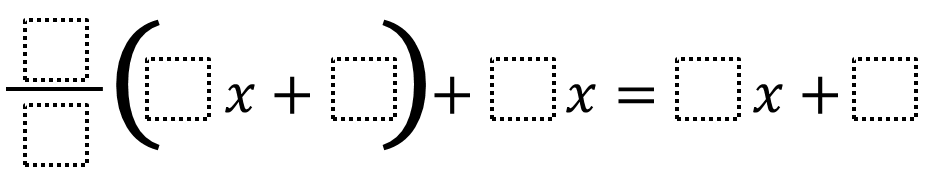# Multi-Step Equations – Positive (or Negative) Solution

Directions: Using the digits 1 to 9, at most one time each, create an equation where x has a positive (or negative) value.### Hint

What makes a solution positive (or negative)?
Where do you want to put the larger (or smaller) numbers?

There are many solutions that would work.

Example that would give a positive solution:
(1/2)(4x + 8) + 6x = 9x + 3 … here, x = 1

Example that would give a negative solutions
(1/3)(6x + 9) + 2x = 5x + 7 … here, x = -4

Another constraint or extension you could add is change the directions to say: “Use the digits 1 to 9, at most one time each, to create an equation where x has a positive (or negative) integer value.”

Source: Daniel Luevanos

## Systems of Equations 1

Directions: Using the integers -9 to 9 at most one time each, fill in the …

1.(1/3)(6x+9)+2x=5x+7
x=-4

2.(1/2)(4x+8)+6x=9x+3
x=1

3.3/6(2x+4)+7x=6x+5
x= 1.5

4.2/6(4x+8)+7x=3x+9
x=1.2

5.1/3(4+8)+2x=2x+8
x=4

6.1/2(5x+6)+3x=4x+9

X=4

7.1/2(-9x(4)=8x(2)

8.(1/2)(4x + 8) + 6x = 9x + 3

which is 1

9.(1/2)(4x + 8) + 6x =9x + 3

which is 1

10.5/3 (6x+3+1x=4x+3
x=-0.28

11.3/4(8x+7)+3x=11x+2

12.1/2(4x-2)+3x=-2x+4
x=5

13.1/2x(2x-1)+4x=-6x+2
x=no solution

14.2/3x-1/6=1/2x+5/6
x=6

15.(1/2)(4x+8)+6x=9x+3
x=1

16.2/1(4x+6)+7x=5x+8

17.(1/2)(4x+9)+6x= 7x+2

18.1/2(5x+6)+3x=4x+9 X=4

19.1/5 (3x + 2) + 4x = 6x + 8
X=6

20.1/5 (3x + 2) + 4x = 6x + 8
X=6

21.3/4m−2(m−1)= 1/4m+5
m=-2

22.1/6(2x+3)+7x=-12+10
x=3

23.1/6(1x+9)+2x=4x+6

x=5

24.25.